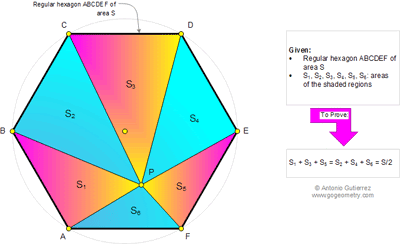## Sunday, February 16, 2014

### Plane Geometry Problem 983. Regular Hexagon, A point and six triangles, Areas

Geometry Problem
Level: Mathematics Education, High School, Honors Geometry, College.

Click the figure below to see the complete problem 9831.Extend AB, EF and CD to form a equilateral triangle LMN with side= a ( see sketch)
Area of LMN= ½.h.a= 1/2a.(h1+h3+h5) => h1+h3+h5=a
Similarly by extend BC, DE and AF to form a equilateral triangle with side= a
We also have h2+h4+h6= a => h1+h3+h5=h2+h4+h6=> S1+S3+S5=S2+S4+S6

1.Correction due to typo error with additional explanation.
Let h1 , h3, h5 are altitudes of triangles with areas equal S1, S3 and S5
Let h2 , h4, h6 are altitudes of triangles with areas equal S2, S4 and S6
Extend AB, EF and CD to form a equilateral triangle LMN with side= a .
Area of LMN= ½.h.a= 1/2a.(h1+h3+h5) => h1+h3+h5=h where h is the altitude of triangle LMN= a.sqrt(3)/2
Similarly by extend BC, DE and AF to form a equilateral triangle with side= a
We also have h2+h4+h6= h => h1+h3+h5=h2+h4+h6
Since all triangles of S1 to S6 have bases with the same value => S1+S3+S5=S2+S4+S6
Peter Tran